﻿ 金属腐蚀区域图像增强算法研究
«上一篇文章快速检索 高级检索

 智能系统学报2019, Vol. 14Issue (2): 385-392  DOI: 10.11992/tis.2017120240

### 引用本文LEI Fang, XIONG Jianbin, ZHANG Lei, et al. Image enhancement algorithm in metal corrosion area[J]. CAAI Transactions on Intelligent Systems, 2019, 14(2): 385-392. DOI: 10.11992/tis.201712024.### 文章历史

1. 广东石油化工学院 计算机与电子信息学院，广东 茂名 525000;
2. 广东技术师范学院 自动化学院，广东 广州 510665;
3. 湖南大学 电气与信息工程学院，湖南 长沙 410082

Image enhancement algorithm in metal corrosion area
LEI Fang 1, XIONG Jianbin 2, ZHANG Lei 1, GUO Siyu 3
1. College of Computer and Electronic Information, Guangdong University of Petrochemical Technology, Maoming 525000, China;
2. School of Automation, Guangdong Polytechnic Normal University, Guangzhou 510665, China;
3. School of Electrical and Information Engineering, Hu’nan University, Changsha 410082, China
Abstract: Considering the images of the metal corrosion areas, the dark details have low contrast, and the illumination is not uniform; meanwhile, the color characteristics need to be preserved. To solve these problems, an approach based on multi-scale detail-adaptive enhancement and homomorphic filtering is proposed on the basis of the HSI model. First, the RGB corrosion image was color-transformed, whereby the hue and saturation components were preserved, and the luminance component was enhanced. Then, wavelet transform was used to implement multi-scale detail-adaptive enhancement, increase the contrast of the detail, and apply block homomorphic filtering, so as to improve the impact of non-uniform illumination. Consequently, the corrosion image was enhanced. Experimental results show that the proposed method can increase the contrast of dark details and improve the overall brightness of the image of the metal corrosion area, ensuring that the color information is undistorted.
Key words: metal corrosion image    HSI model    multi-scale    detail-adaptive enhancement    block homomorphic filtering

1 本文方法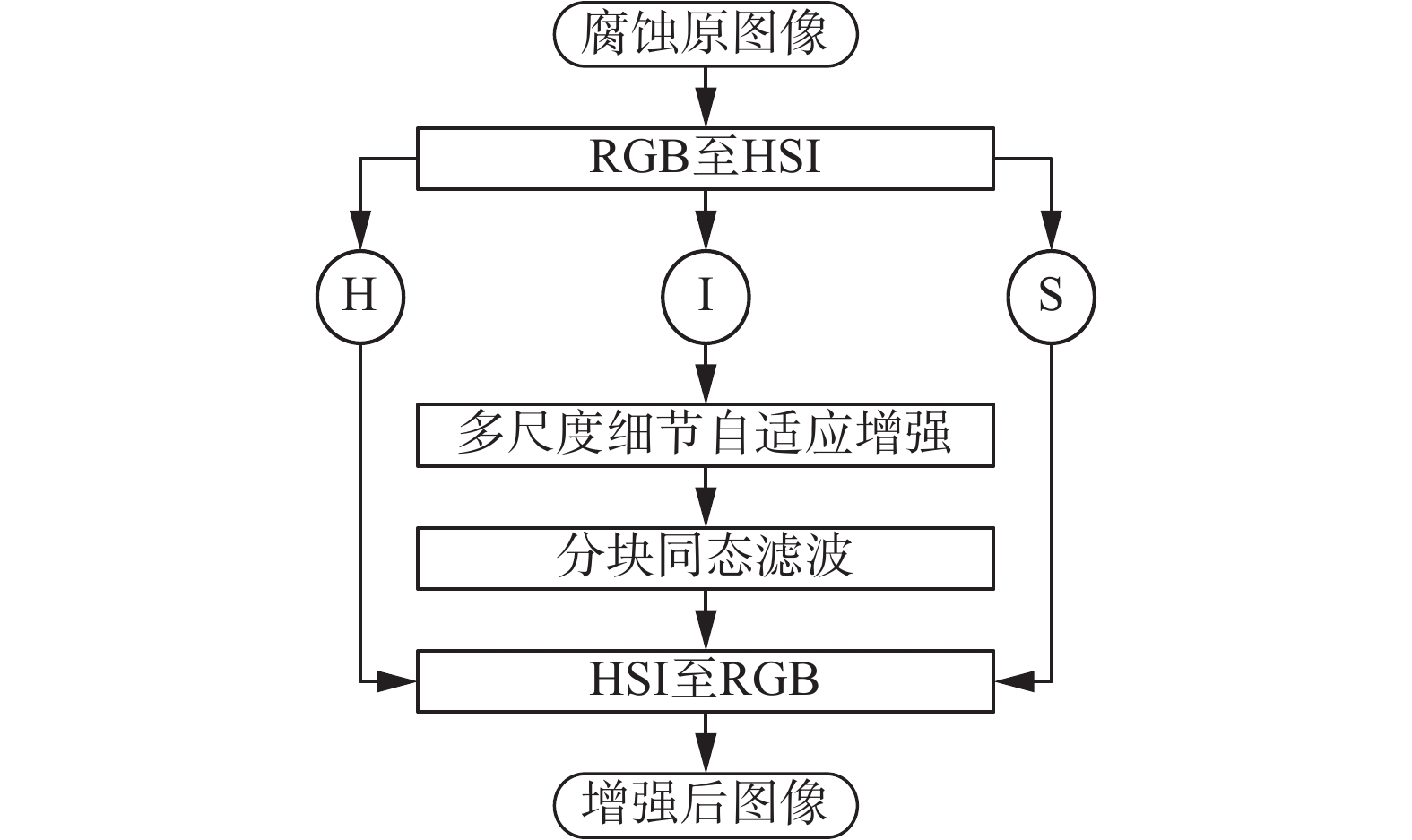Download: 图 1 提出方法流程图 Fig. 1 Flow chart of proposed algorithm
1.1 腐蚀处理中的HSI模型应用

RGB模型转换为HSI模型的公式如式(1)：

 \left\{ \begin{aligned} & {{H}} = \left\{ {\begin{array}{l} {\;\theta ,\quad {{B}} \leqslant {{G}}}\\ {360 - \theta ,\quad {{B}} > {{G}}} \end{array}} \right.\\ & {{S}} = 1 - \frac{3}{{({{R}} + {{G}} + {{B}})}}[\min ({{R}},{{G}},{{B}})]\\ & {{I}} = \frac{1}{3}({{R}} + {{G}} + {{B}})\\ & \theta = \arccos \left\{ {\frac{{\displaystyle\frac{1}{2}[({{R}} - {{G}}) + ({{R}} - {{B}})]}}{{{{[{{({{R}} -{{G}})}^2} + ({{R}} - {{B}})({{G}} - {{B}})]}^{1/2}}}}} \right\} \end{aligned} \right. (1)

 \left\{ \begin{aligned} & \left\{ \begin{aligned} & {{B}} = {{I}}(1 - {{S}})\\ & {{R}} = {{I}}\left[ {1 + \frac{{{{S}}\cos {{H}}}}{{\cos ({{60}^ \circ } - {{H}})}}} \right]\\ & {{G}} = 3{{I}} - ({{B}} + {{R}}) \end{aligned} \right.\quad ,\quad \quad {0^ \circ } \leqslant {{H}} < {120^ \circ }\\ & \left\{ \begin{aligned} & {{R}} = {{I}}(1 - {{S}})\\ & {{G}} = {{I}}\left[ {1 + \frac{{{{S}}\cos ({{H}} - {{120}^ \circ }}}{{\cos ({{180}^ \circ } - {{H}})}}} \right]\\ & {{B}} = 3{{I}} - ({{G}} + {{R}}) \end{aligned} \right.,\quad \quad {120^ \circ } \leqslant {{H}} < {240^ \circ }\\ & \left\{ \begin{aligned} &{{R}} = 3{{I}} - ({{G}} + {{B}})\\ & {{B}} = {{I}}\left[ {1 + \frac{{{{S}}\cos ({{H}} - {{240}^ \circ }}}{{\cos ({{360}^ \circ } - {{H}})}}} \right]\\ & {{G}} = {{I}}(1 - {{S}}) \end{aligned} \right.,\quad \quad {120^ \circ } \leqslant {{H}} < {240^ \circ } \end{aligned} \right. (2)
1.2 多尺度细节自适应增强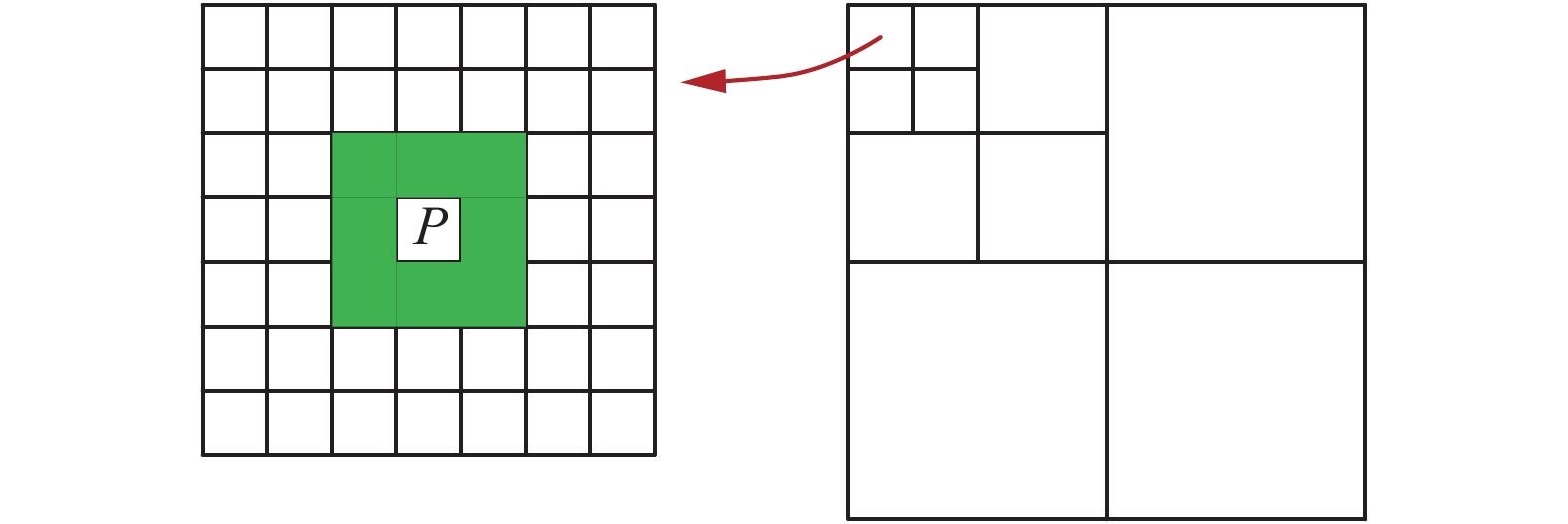Download: 图 2 近似分量中像素点P的3×3邻域 Fig. 2 3×3 neighbor of pixel P in similar component

1) 计算图像 $f(x,y)$ 的最大值 ${f_{\max }}$ 和最小值 ${f_{\min }}$

2) 对每级分解图像近似分量进行非线性滤波， $f({x_i},{y_i}) = (\max ({x_i}) - \min ({x_i}))/({f_{\max }} - {f_{\min }})$ 。其中 $\max ({x_i})$ $\min ({x_i})$ 为滤波过程中心像素邻域内的最大值和最小值。

3) 计算第 $i$ 级图像近似分量的全局对比度，其值为 ${C_{\rm globali}} = {c_0} \cdot {L_{\rm sumi}}/num({f_i})$ 。其中， ${L_{\rm sumi}}$ 为非线性滤波输出图像的像素值之和， ${\rm num}({f_i})$ 为图像的像素点个数， ${c_0}$ 为对比度调节因子，一般取值范围为1.0~1.1。

4) 引入第 $i$ 级全局对比度权重因子 ${K_i}$ ，其中， ${K_{{i}}} = \displaystyle\frac{1}{2}\log (\frac{1}{{{C_{\rm globali}}}} + 1)$ 。将其与细节分量的系数相乘，调整细节图像。

5) 在调节细节分量的同时，也可根据实际需要将近似分量乘以亮度因子 ${L_0}$ ，其一般取值范围为1.0~1.2。

6) 根据自适应细节增强系数，重构图像， $g(x,y) =$ ${L_0} \cdot L{L_3} + \displaystyle\sum\limits_{i = 3}^1 {{K_i} \cdot (L{H_i} + H{L_i} + H{H_i})}$

1.3 分块同态滤波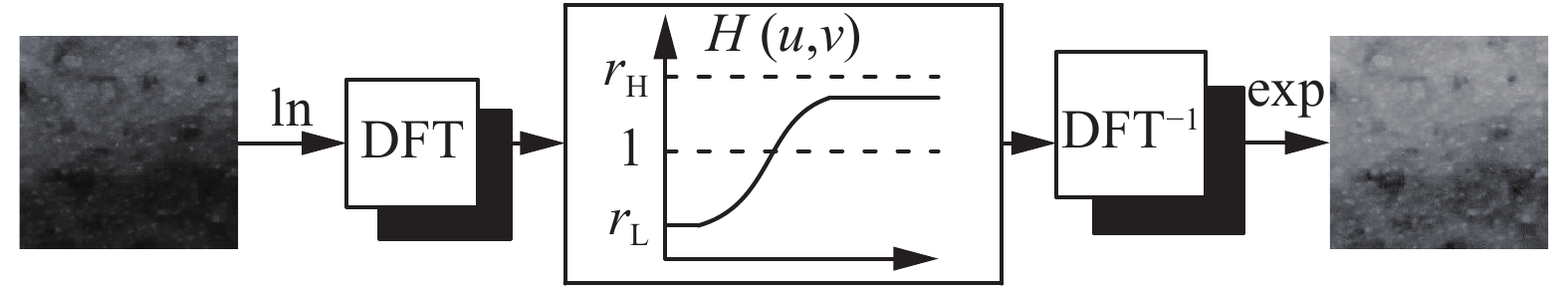Download: 图 3 同态滤波流程图 Fig. 3 Flow chart of homomorphic

 $H(u,v) = ({r_H} - {r_L})[1 - {{\rm e}^{ - c{{[\frac{{{D_0}^n}}{{{{(D(u,v))}^n}}}]}^2}}}] + {r_L}$ (3)

 $D(u,v) = \sqrt {{{(u - M/2)}^2} + {{(v - N/2)}^2}}$ (4)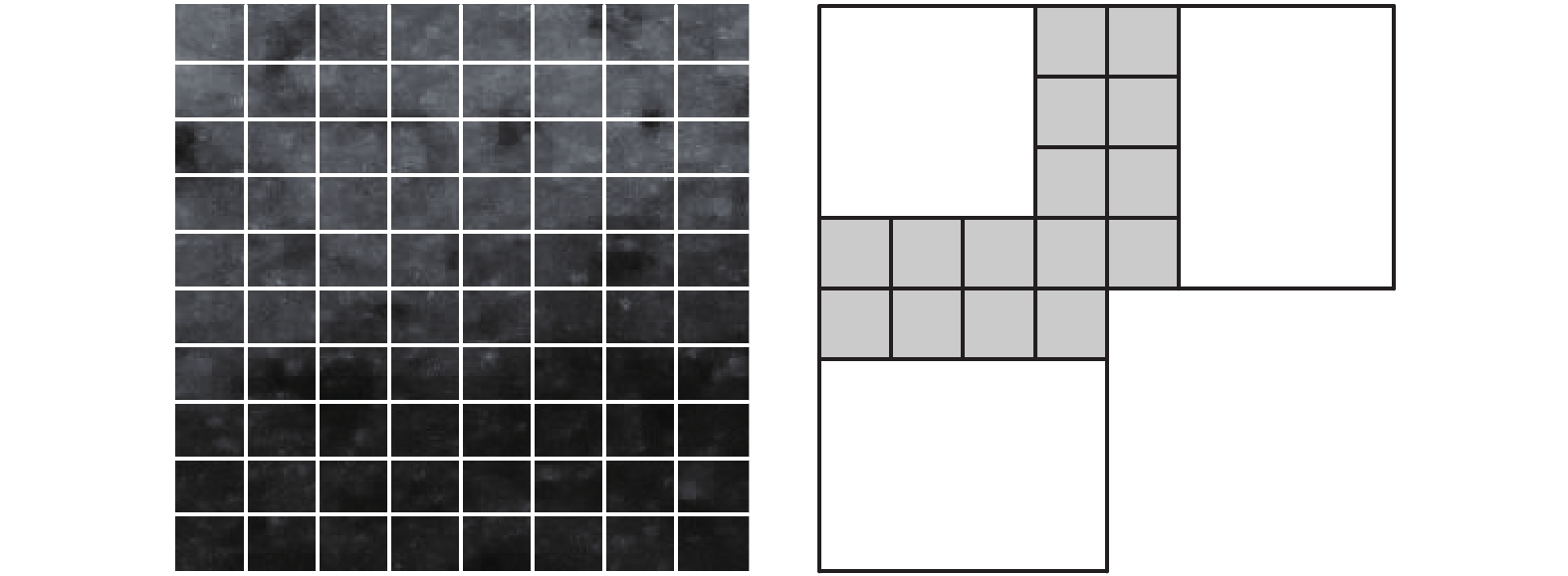Download: 图 4 同态滤波及其块效应 Fig. 4 Homomorphic and block effect
 $L(m,n) = \frac{{\sum\limits_{i = 0}^{n_0} {L(m,n - i)} + \sum\limits_{i = 0}^{n_0 - 1} {R(m,i)} }}{N}\quad 0 \leqslant m \leqslant 7,n = 7$ (5)
 $R(m,n) = \frac{{\sum\limits_{i = 0}^{n_0} {L(m,7 - i)} + \sum\limits_{i = 0}^{n_0} {R(m,n + i)} }}{N}\quad 0 \leqslant m \leqslant 7,n = 0$ (6)
 $U(m,n) = \frac{{\sum\limits_{i = 0}^{m_0} {U(m - i,n)} + \sum\limits_{i = 0}^{m_0 - 1} {D(i,n)} }}{M}\quad 0 \leqslant n \leqslant 7,m = 7$ (7)
 $D(m,n) = \frac{{\sum\limits_{i = 0}^{m_0 - 1} {U(7 - i,n)} + \sum\limits_{i = 0}^{m_0} {D(m + i,n)} }}{M}\quad 0 \leqslant n \leqslant 7,m = 0$ (8)

2 实验结果与分析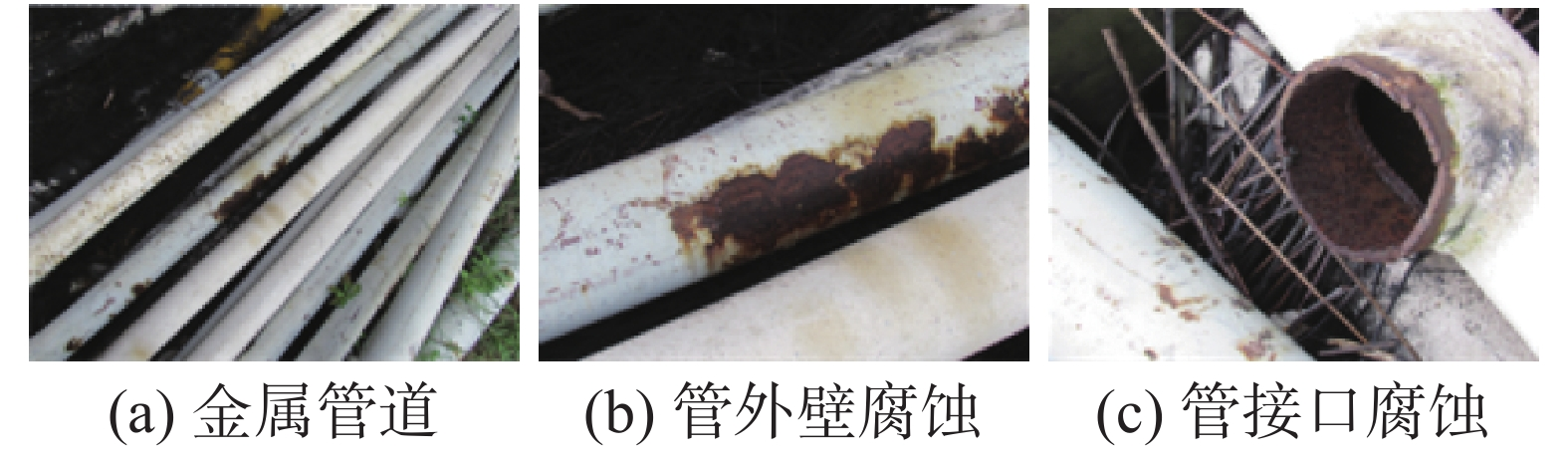Download: 图 5 自然环境下受到腐蚀的金属管道 Fig. 5 Corrosion metal pipeline in natural environment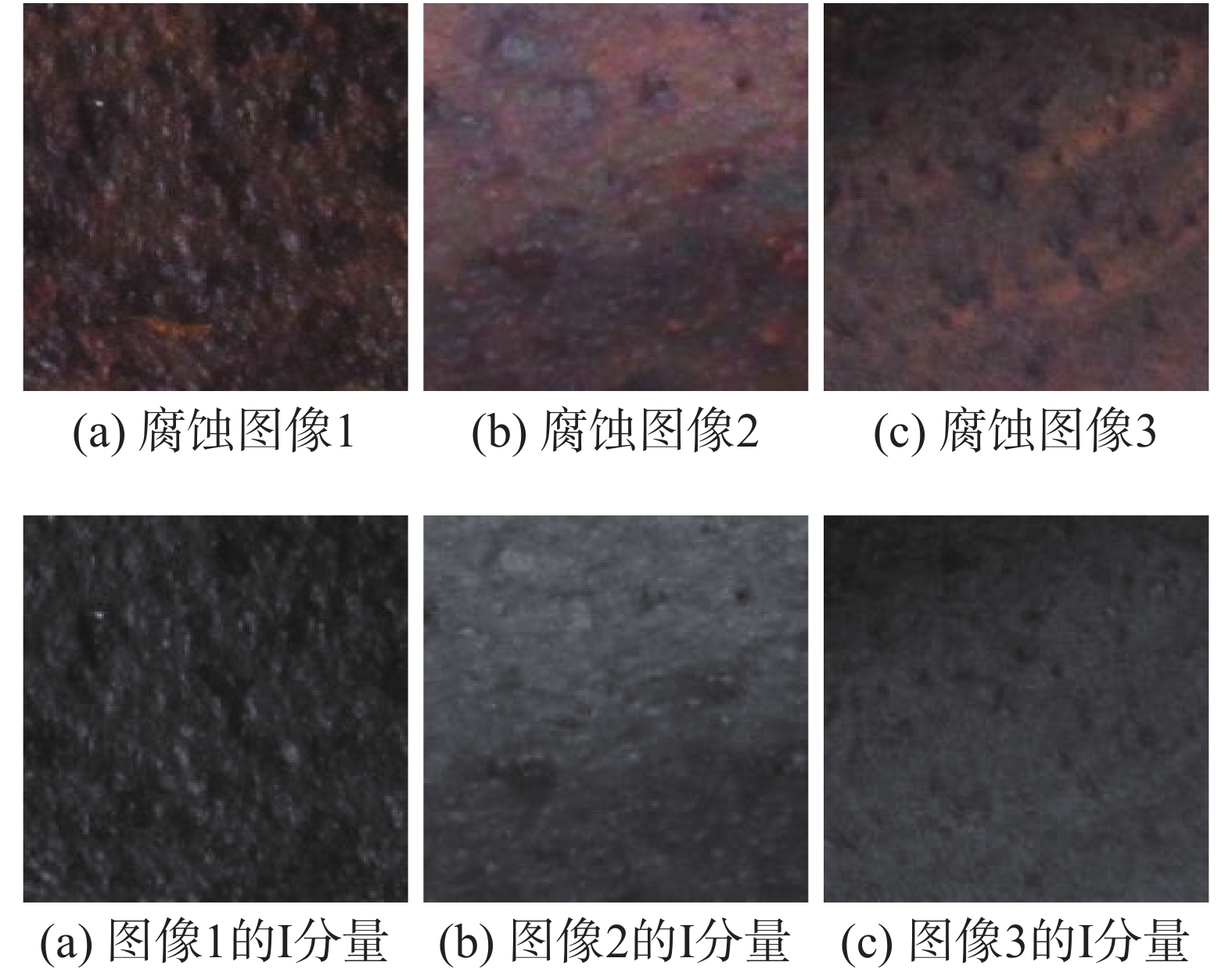Download: 图 6 腐蚀原图像及I分量 Fig. 6 Corrosion image and I component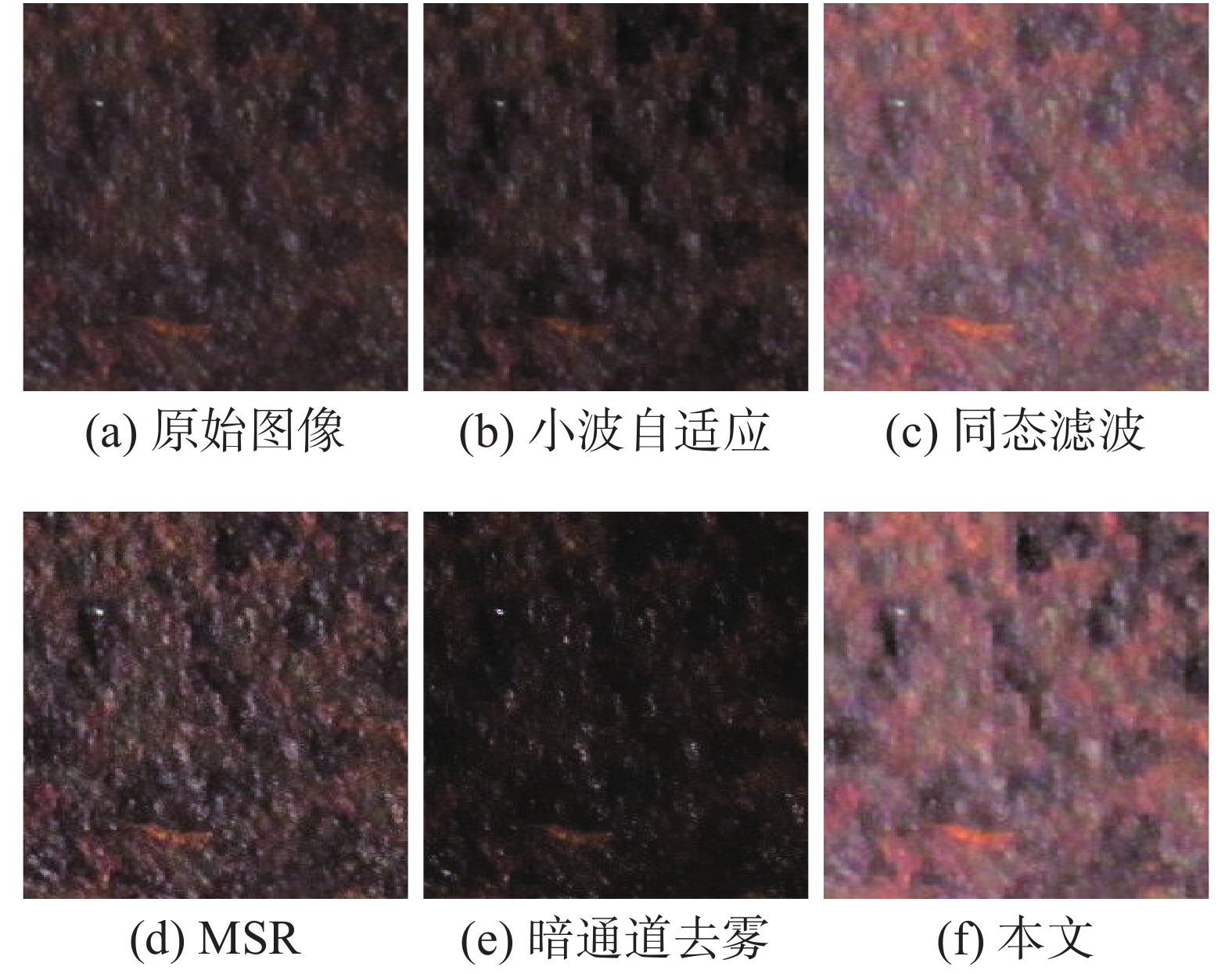Download: 图 7 腐蚀图像1增强效果比较 Fig. 7 Enhancement results comparison in corrosion image 1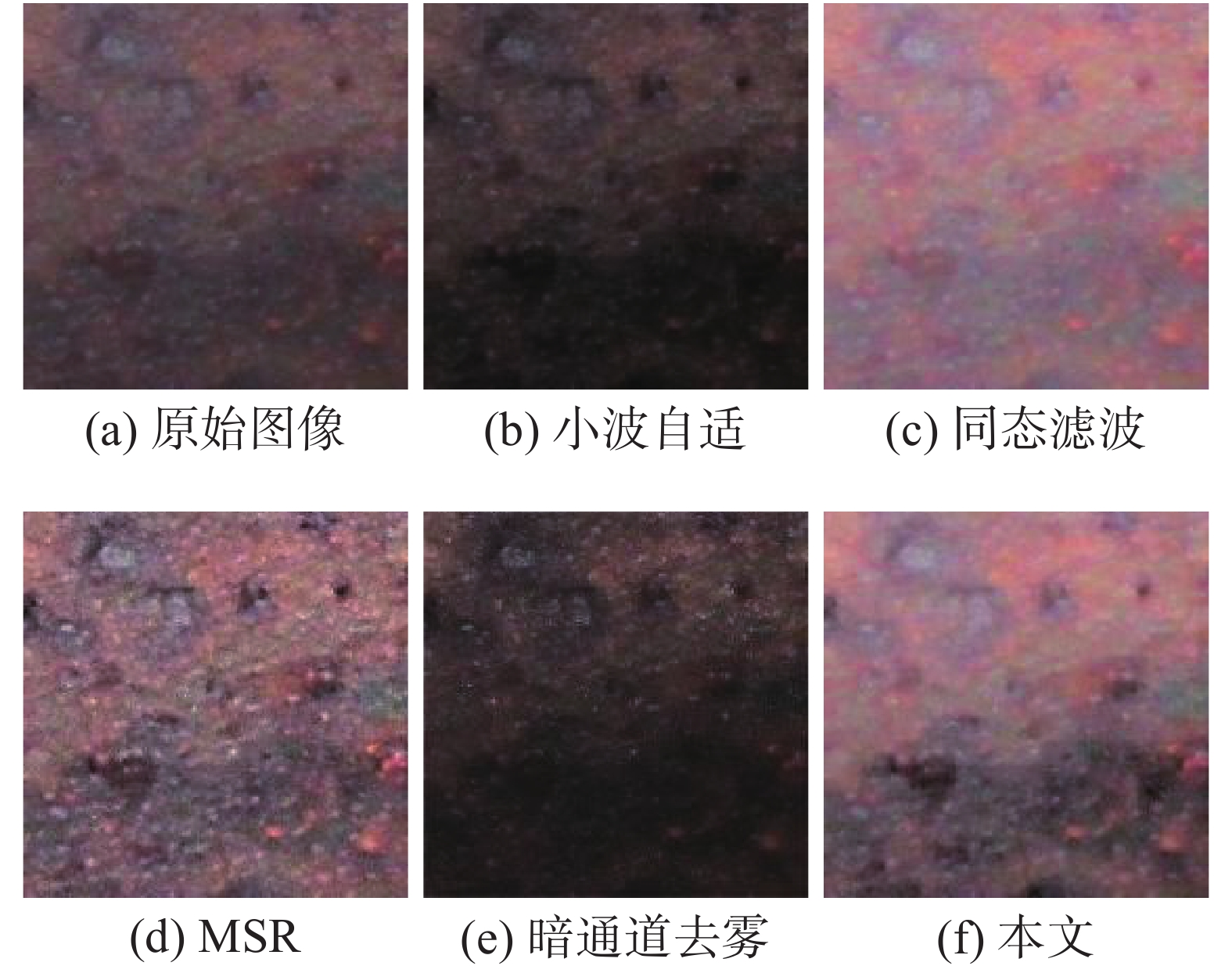Download: 图 8 腐蚀图像2增强效果比较 Fig. 8 Enhancement results comparison in corrosion image 2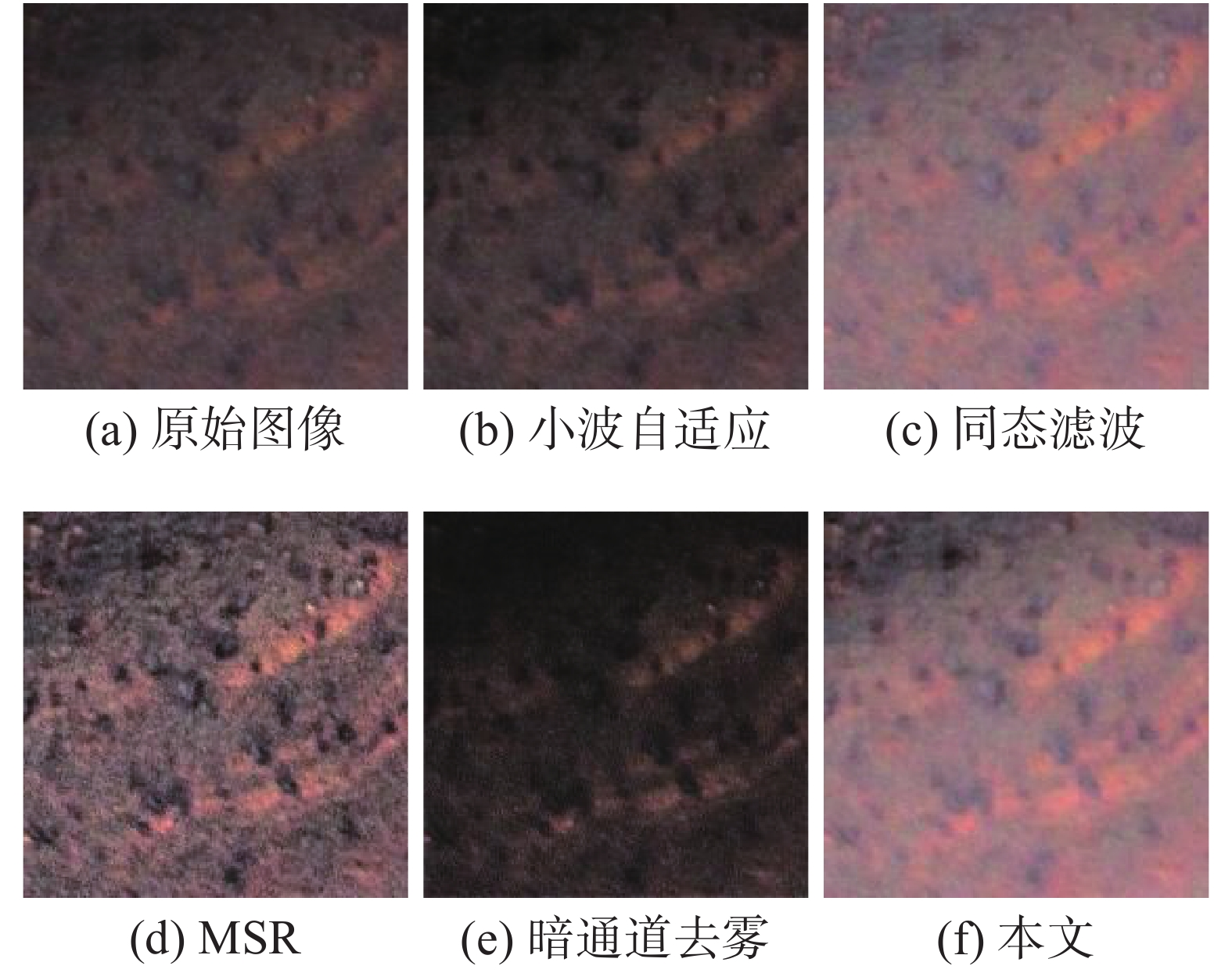Download: 图 9 腐蚀图像3增强效果比较 Fig. 9 Enhancement results comparison in corrosion image 3表 1 各图像亮度分量的信息熵 Tab.1 Entropy of intensity component in each image表 2 图像集的信息熵指标对比 Tab.2 Entropy index comparison in image set

3 结束语

  MEDEIROS F N S, RAMALHO G L B, BENTO M P, et al. On the evaluation of texture and color features for nondestructive corrosion detection[J]. EURASIP journal on advances in signal processing, 2010, 2010: 817473. DOI:10.1155/2010/817473 (0)  纪钢, 彭丽丽, 王平. 基于腐蚀产物颜色分析的材料腐蚀程度评定方法[J]. 重庆理工大学学报 (自然科学版), 2012, 26(7): 69-73. JI Gang, PENG Lili, WANG Ping. Research on the method for evaluation of material corrosion degree based on color analysis of corrosion products[J]. Journal of Chongqing University of Technology (natural science), 2012, 26(7): 69-73. (0)  IDRIS S A, JAFER F A. Image enhancement filter evaluation on corrosion visual inspection[M]//SULAIMAN H A, OTHMAN M A, OTHMAN M F I, et al. Advanced Computer and Communication Engineering Technology. Cham: Springer, 2015: 651–659. (0)  HE Kaiming, SUN Jian, TANG Xiaoou. Guided image filtering[J]. IEEE transactions on pattern analysis and machine intelligence, 2013, 35(6): 1397-1409. DOI:10.1109/TPAMI.2012.213 (0)  PIDAPARTI R M, HINDERLITER B, MASKEY D. Evaluation of corrosion growth on SS304 based on textural and color features from image analysis[J]. ISRN corrosion, 2013, 2013: 376823. (0)  ROBERGE P R. Corrosion inspection and monitoring[M]. Hoboken, N.J.: John Wiley & Sons, 2007: 1–50. (0)  毛东月, 谢正祥, 贺向前, 等. 自适应双向保带宽对数变换及低照度图像增强[J]. 中国图象图形学报, 2017, 22(10): 1356-1363. MAO Dongyue, XIE Zhengxiang, HE Xiangqian, et al. Adaptive bilateral logarithm transformation with bandwidth preserving and low-illumination image enhancement[J]. Journal of image and graphics, 2017, 22(10): 1356-1363. (0)  SINGH K, KAPOOR R. Image enhancement using exposure based sub image histogram equalization[J]. Pattern recognition letters, 2014, 36: 10-14. DOI:10.1016/j.patrec.2013.08.024 (0)  汪林林, 余梅, 安超. 模糊多尺度Retinex彩色图像增强[J]. 计算机工程与应用, 2012, 48(7): 1343-1346. WANG Linlin, YU Mei, AN Chao. Color image enhancement based on fuzzy multiple-scale Retinex[J]. Computer engineering and applications, 2012, 48(7): 1343-1346. (0)  汪荣贵, 朱静, 杨万挺, 等. 基于照度分割的局部多尺度Retinex算法[J]. 电子学报, 2010, 38(5): 1181-1186. WANG Ronggui, ZHU Jing, YANG Wanting, et al. An improved local multi-scale retinex algorithm based on illuminance image segmentation[J]. Acta electronica sinica, 2010, 38(5): 1181-1186. (0)  汪荣贵, 周良, 张新龙, 等. 基于Retinex的JPEG图像增强新方法[J]. 中国图象图形学报, 2011, 16(12): 2124-2132. WANG Ronggui, ZHOU Liang, ZHANG Xinlong, et al. Novel JPEG image enhancement method based on Retinex theory[J]. Journal of image and graphics, 2011, 16(12): 2124-2132. DOI:10.11834/jig.20111205 (0)  XIAO Limei, LI Ce, WU Zongze, et al. An enhancement method for X-ray image via fuzzy noise removal and homomorphic filtering[J]. Neurocomputing, 2016, 195: 56-64. DOI:10.1016/j.neucom.2015.08.113 (0)  张亚飞, 谢明鸿. 基于分块DCT同态滤波的彩色图像增强算法[J]. 计算机工程与设计, 2013, 34(5): 1752-1756. ZHANG Yafei, XIE Minghong. Block-DCT based homomorphic filtering algorithm for color image enhancement[J]. Computer engineering and design, 2013, 34(5): 1752-1756. DOI:10.3969/j.issn.1000-7024.2013.05.045 (0)  马金祥, 范新南, 吴志祥, 等. 暗通道先验的大坝水下裂缝图像增强算法[J]. 中国图象图形学报, 2016, 21(12): 1574-1584. MA Jinxiang, FAN Xinnan, WU Zhixiang, et al. Underwater dam crack image enhancement algorithm based on improved dark channel prior[J]. Journal of image and graphics, 2016, 21(12): 1574-1584. DOI:10.11834/jig.20161202 (0)  王建新, 张有会, 王志巍, 等. 基于HSI颜色空间的单幅图像去雾算法[J]. 计算机应用, 2014, 34(10): 2990-2995. WANG Jianxin, ZHANG Youhui, WANG Zhiwei, et al. Single image defogging algorithm based on HSI color space[J]. Journal of computer applications, 2014, 34(10): 2990-2995. DOI:10.11772/j.issn.1001-9081.2014.10.2990 (0)  秦绪佳, 王慧玲, 杜轶诚, 等. HSV色彩空间的Retinex结构光图像增强算法[J]. 计算机辅助设计与图形学学报, 2013, 25(4): 488-493. QIN Xujia, WANG Huiling, DU Yicheng, et al. Structured light image enhancement algorithm based on retinex in HSV color space[J]. Journal of computer-aided design & computer graphics, 2013, 25(4): 488-493. DOI:10.3969/j.issn.1003-9775.2013.04.008 (0)  HE Kaiming, SUN Jian, TANG Xiaoou. Single image haze removal using dark channel prior[J]. IEEE transactions on pattern analysis and machine intelligence, 2011, 33(12): 2341-2353. DOI:10.1109/TPAMI.2010.168 (0)  孙慧贤, 罗飞路, 张玉华. 基于小波变换和同态滤波的内窥图像增强算法[J]. 探测与控制学报, 2008, 30(5): 68-72. SUN Huixian, LUO Feilu, ZHANG Yuhua. A new homomorphic filtering based on wavelet transform for endoscope image enhancement[J]. Journal of detection & control, 2008, 30(5): 68-72. DOI:10.3969/j.issn.1008-1194.2008.05.019 (0)  徐涛, 李冠章. 基于小波变换的彩色图像自适应细节增强算法[J]. 计算机应用与软件, 2011, 28(3): 240-242, 295. XU Tao, LI Guanzhang. Adaptive detail enhancement algorithm of colour image based on wavelet transform[J]. Computer applications and software, 2011, 28(3): 240-242, 295. DOI:10.3969/j.issn.1000-386X.2011.03.073 (0)  冈萨雷斯R C, 伍兹R E. 数字图像处理[M]. 阮秋琦, 译. 2版. 北京: 电子工业出版社, 2003. (0)  TONG Hanghang, LI Mingjing, ZHANG Hongjiang, et al. Blur detection for digital images using wavelet transform[C]//Proceedings of 2004 IEEE International Conference on Multimedia and Expo (ICME). Taipei, Taiwan, 2004. (0)  肖俊, 宋寿鹏, 丁丽娟. 空域同态滤波算法研究[J]. 中国图象图形学报, 2008, 13(12): 2302-2306. XIAO Jun, SONG Shoupeng, DING Lijuan. Research on the fast algorithm of spatial homomorphic filtering[J]. Journal of image and graphics, 2008, 13(12): 2302-2306. DOI:10.11834/jig.20081209 (0)  赵晓丽, 孙宪坤. 基于视觉特性的彩色图像增强算法研究[J]. 计算机工程与设计, 2009, 30(19): 4458-4460. ZHAO Xiaoli, SUN Xiankun. Color image enhancement algorithm based on human visual system[J]. Computer engineering and design, 2009, 30(19): 4458-4460. (0)  周西柳, 章洁. 基于聚类余弦变换的图像增强算法研究[J]. 计算机仿真, 2012, 29(2): 216-219. ZHOU Xiliu, ZHANG Jie. Image blur based on DCT and clustering algorithm[J]. Computer simulation, 2012, 29(2): 216-219. DOI:10.3969/j.issn.1006-9348.2012.02.050 (0)